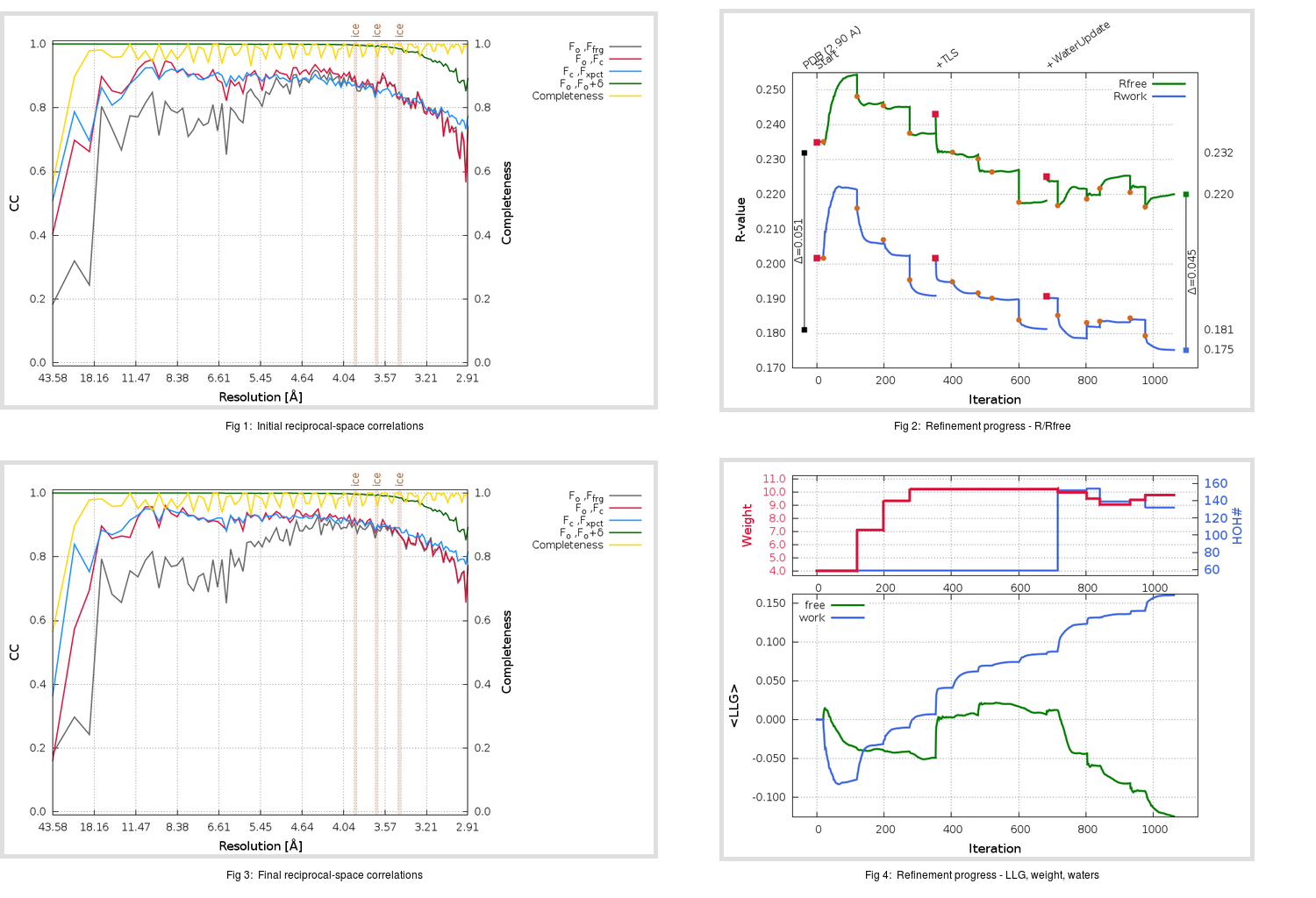Content:

```    Diffraction limits & principal axes of ellipsoid fitted to diffraction cut-off surface:
2.895         1.0000   0.0000   0.0000       0.894 a* - 0.447 b*
2.895         0.0000   1.0000   0.0000       b*
2.814         0.0000   0.0000   1.0000       c*
```

## Deposited

` `
 Date deposited Date data collection Resolution R, Rfree 20141030 20140213 2.90 0.1790 0.2320

Molprobity (CCP4 7.0 version) summary:

```Ramachandran outliers =   0.38 %
favored =  94.75 %
Rotamer outliers      =   3.02 %
C-beta deviations     =     0
Clashscore            =   4.24
RMS(bonds)            =   0.0102
RMS(angles)           =   1.47
MolProbity score      =   1.93
Resolution            =   2.90
R-work                =   0.1790
R-free                =   0.2320
```

```Number of waters      =    59

<B> (all atoms) =   58.90 ( sd =   15.31 ) for       4416 non-hydrogen atoms
<B>   (protein) =   57.61 ( sd =   14.07 ) for       4073 non-hydrogen atoms
<B>     (water) =   43.18 ( sd =    6.86 ) for         59 non-hydrogen atoms
<B>    (others) =   80.70 ( sd =   15.66 ) for        284 non-hydrogen atoms

B min/max       (all non-hydrogen atoms) =   26.56 /  138.98
B min/max   (protein non-hydrogen atoms) =   34.21 /  122.85
B min/max     (water non-hydrogen atoms) =   26.56 /   55.11
B min/max     (other non-hydrogen atoms) =   48.54 /  138.98
```

## BUSTER (re-)refinement

` `

Molprobity (CCP4 7.0 version) summary:

```Ramachandran outliers =   0.38 %
favored =  96.44 %
Rotamer outliers      =   3.72 %
C-beta deviations     =     0
Clashscore            =   3.06
RMS(bonds)            =   0.0128
RMS(angles)           =   1.61
MolProbity score      =   1.77
Resolution            =   2.90
R-work                =   0.1753
R-free                =   0.2200
```

```Number of waters      =   132

<B> (all atoms) =   64.30 ( sd =   14.47 ) for       4489 non-hydrogen atoms
<B>   (protein) =   62.39 ( sd =   11.53 ) for       4073 non-hydrogen atoms
<B>     (water) =   56.16 ( sd =   12.66 ) for        132 non-hydrogen atoms
<B>    (others) =   95.46 ( sd =   16.55 ) for        284 non-hydrogen atoms

B min/max       (all non-hydrogen atoms) =   31.12 /  135.15
B min/max   (protein non-hydrogen atoms) =   42.43 /  120.58
B min/max     (water non-hydrogen atoms) =   31.12 /  128.27
B min/max     (other non-hydrogen atoms) =   60.54 /  135.15
```

Refinement progression:Results:

` `
 File Remark 4WTG_aB_refine.01_03_refine.pdb.gz exact refinement commands are in header 4WTG_aB_refine.01_03_refine.mtz.gz including original deposited data and several re-refinement map coefficients 4WTG_aB_refine.01_03_BUSTER_model.cif.gz including any non-standard compound restraints 4WTG_aB_refine.01_03_BUSTER_refln.cif.gz